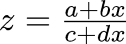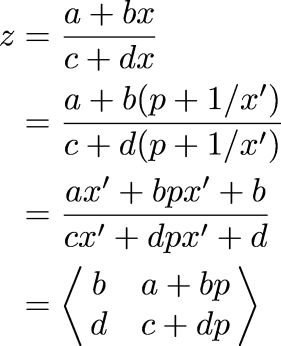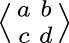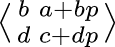Next Continued Fraction Arithmetic 28

# Input

• Let's suppose thatrequests an input from x

• And the next term from x turns out to be p

• As a result, x has changed into x'

• And z now knows that x = p + 1/x'• Thus ifrequests an input and gets p, it turns into• "Ingestion"

 Next Back